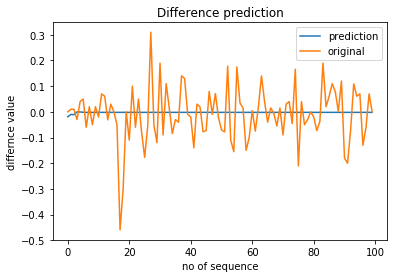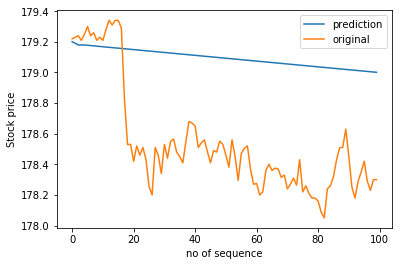# Error in Forecasting Stock price using LSTM in Keras

I created an LSTM model for intraday stock predictions. I took the training data with the shape of (290, 4). I did all the preprocessing like Normalize the data, taking the difference, taking window size of 4.

This is a sample of my input data.

``````X = array([[0, 0, 0, 0],
[array([ 0.19]), 0, 0, 0],
[array([-0.35]), array([ 0.19]), 0, 0],
...,
[array([ 0.11]), array([-0.02]), array([-0.13]), array([-0.09])],
[array([-0.02]), array([ 0.11]), array([-0.02]), array([-0.13])],
[array([ 0.07]), array([-0.02]), array([ 0.11]), array([-0.02])]], dtype=object)

y = array([[array([ 0.19])],
[array([-0.35])],
[array([-0.025])],
.....,
[array([-0.02])],
[array([ 0.07])],
[array([-0.04])]], dtype=object)
``````

Note: I am giving as well as predicting the difference value. So input value is between range (-0.5,0.5)

Here is my Keras LSTM model :

``````dim_in = 4
dim_out = 1

return_sequences=True,
units=6))

model.compile(loss = 'mse', optimizer = 'rmsprop')

for i in range(300):
#print("Completed :",i+1,"/",300, "Steps")
model.fit(X, y, epochs=1, batch_size=1, verbose=2, shuffle=False)
model.reset_states()
``````

I am feeding the last sequence value of shape=(1,4) and predict the output.
This is my prediction :

``````base_value = df.iloc['Close']
prediction = []
orig_pred = []
input_data = np.copy(test[0,:])
input_data = input_data.reshape(len(input_data),1)
for i in range(100):
inp = input_data[i:,:]
inp = inp.reshape(1,1,inp.shape)
y = model.predict(inp)
orig_pred.append(y)
input_data = np.insert(input_data,[i+4],y, axis=0)
base_value = base_value  + y
prediction_apple.append(base_value)
sqrt(mean_squared_error(test_output, orig_pred))`
``````

RMSE = 0.10592485833344527

Here is the predicted difference value and predicted stock price visualizations.fig:1 -> This is the LSTM predictionfig:2 -> This is the Stock prediction

I am not sure why it is predicting the same output value after 10 iterations. Maybe it is the vanishing gradient problem or I am feeding fewer input data(290 approx) or problem in the model architecture. I am not sure.